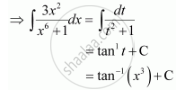Share

# Integrate the Functions (3x^2)/(X^6 + 1) - CBSE (Commerce) Class 12 - Mathematics

ConceptIntegrals of Some Particular Functions

#### Question

Integrate the functions (3x^2)/(x^6 + 1)

#### Solution

Let x3 = t

∴ 3x2 dx = dtIs there an error in this question or solution?

#### Video TutorialsVIEW ALL 

Solution Integrate the Functions (3x^2)/(X^6 + 1) Concept: Integrals of Some Particular Functions.
S